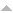## Publications

Export 6 results:
Sort by: Author [ Title] Type Year
S
Chaari, S., F. Cipriano, Soumaya Gheryani, and H. Ouerdiane. "Sanov's Theorem for White Noise Distributions and Application to the Gibbs Conditioning Principle." ACTA APPLICANDAE MATHEMATICAE. 104 (2008): 313-324. Abstract

{We consider a positive distribution Phi such that Phi defines a probability measure mu = mu Phi on the dual of some real nuclear Frechet space. A large deviation principle is proved for the family \{mu(n), n >= 1\} where mu(n) denotes the image measure of the product measure mu(n)(Phi) under the empirical distribution function L(n). Here the rate function I is defined on the space F(theta)'(N')(+) and agrees with the relative entropy function (H) over tilde (Psi/Phi). As an application, we cite the Gibbs conditioning principle which describes the limiting behaviour as n tends to infinity of the law of k tagged particles Y(1),...,Y(k) under the constraint that L(n)(Y) belongs to some subset A(0).}

Chemetov, N. V., F. Cipriano, and S. Gavrilyuk. "Shallow water model for lakes with friction and penetration." MATHEMATICAL METHODS IN THE APPLIED SCIENCES. 33 (2010): 687-703. Abstract

{We deduce a shallow water model, describing the motion of the fluid in a lake, assuming inflow-outflow effects across the bottom. This model arises from the asymptotic analysis of the 3D dimensional Navier-Stokes equations. We prove the global in time existence result for this model in a bounded domain taking the nonlinear slip/friction boundary conditions to describe the inflows and outflows of the porous coast and the rivers. The solvability is shown in the class of solutions with L(p)-bounded vorticity for any given p is an element of (1, infinity). Copyright (C) 2009 John Wiley & Sons, Ltd.}

Mendes, Vilela R., and Fernanda Cipriano. "A stochastic representation for the Poisson-Vlasov equation." COMMUNICATIONS IN NONLINEAR SCIENCE AND NUMERICAL SIMULATION. 13 (2008): 221-226. Abstract

{A stochastic representation for the solutions of the Poisson-Vlasov equation is obtained. The representation involves both an exponential and a branching process. The stochastic representation, besides providing an alternative existence proof and an intuitive characterization of the solutions, may also be used to obtain an intrinsic definition of the fluctuations. (c) 2007 Elsevier B.V. All rights reserved.}

Cipriano, F., Ouerdiane Vilela Mendes H. R. "Stochastic Solution of a KPP-Type Nonlinear Fractional Differential Equation." Fractional Calculus and Applied Analysis. 12 (2009): 47-56. AbstractWebsite

Mathematics Subject Classification: 26A33, 76M35, 82B31A stochastic solution is constructed for a fractional generalization of the KPP (Kolmogorov, Petrovskii, Piskunov) equation. The solution uses a fractional generalization of the branching exponential process and propagation processes which are spectral integrals of Levy processes.

Cipriano, F. "A Stochastic variational principle for Burgers equation and its symmetries." STOCHASTIC ANALYSIS AND MATHEMATICAL PHYSICS II. Ed. R. } {Rebolledo. {TRENDS IN MATHEMATICS}. VIADUKSTRASSE 40-44, PO BOX 133, CH-4010 BASEL, SWITZERLAND: Catedra Presiden Analis Cualitat Sistemas Dinam Cuant; Univ Catol, Direcc Invest Postgrado; FONDECYT; ICCTICONICYT Exchange Programme, 2003. {29-46}. Abstract

{A stochastic variational principle for the classical Burgers equation is established. A solution of this equation can be considered as the velocity field of a stochastic process which is a minimum of an energy functional. A family of stochastic constants of the motion, determined in terms of the probability distribution of that process, yields the complete list of symmetries of the Burgers equation.}

Chaari, S., F. Cipriano, H. - H. Kuo, and H. Ouerdiane. "Surface measures on the dual space of the Schwartz space." Commun. Stoch. Anal.. 4 (2010): 467-480. Abstract

n/a